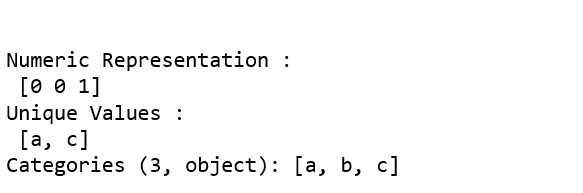# Python | Pandas.factorize()

pandas.factorize() method helps to get the numeric representation of an array by identifying distinct values. This method is available as both `pandas.factorize()` and `Series.factorize()`.

Parameters:
values : 1D sequence.
sort : [bool, Default is False] Sort uniques and shuffle labels.
na_sentinel : [ int, default -1] Missing Values to mark ‘not found’.

Return: Numeric representation of array

Code: Explaining the working of factorize() method

 `# importing libraries ` `import` `numpy as np ` `import` `pandas as pd ` `from` `pandas.api.types ``import` `CategoricalDtype ` ` `  `labels, uniques ``=` `pd.factorize([``'b'``, ``'d'``, ``'d'``, ``'c'``, ``'a'``, ``'c'``, ``'a'``, ``'b'``]) ` ` `  `print``(``"Numeric Representation : \n"``, labels) ` `print``(``"Unique Values : \n"``, uniques) ``# sorting the numerics ` `label1, unique1 ``=` `pd.factorize([``'b'``, ``'d'``, ``'d'``, ``'c'``, ``'a'``, ``'c'``, ``'a'``, ``'b'``],  ` `                                                           ``sort ``=` `True``) ` ` `  `print``(``"\n\nNumeric Representation : \n"``, label1) ` `print``(``"Unique Values : \n"``, unique1) ``# Missing values indicated ` `label2, unique2 ``=` `pd.factorize([``'b'``, ``None``, ``'d'``, ``'c'``, ``None``, ``'a'``, ],  ` `                                              ``na_sentinel ``=` `-``101``) ` ` `  `print``(``"\n\nNumeric Representation : \n"``, label2) ` `print``(``"Unique Values : \n"``, unique2) ``# When factorizing pandas object; unique will differ  ` `a ``=` `pd.Categorical([``'a'``, ``'a'``, ``'c'``], categories ``=``[``'a'``, ``'b'``, ``'c'``]) ` ` `  `label3, unique3 ``=` `pd.factorize(a) ` ` `  `print``(``"\n\nNumeric Representation : \n"``, label3) ` `print``(``"Unique Values : \n"``, unique3) `My Personal Notes arrow_drop_upIf you like GeeksforGeeks and would like to contribute, you can also write an article using contribute.geeksforgeeks.org or mail your article to contribute@geeksforgeeks.org. See your article appearing on the GeeksforGeeks main page and help other Geeks.

Please Improve this article if you find anything incorrect by clicking on the "Improve Article" button below.

Article Tags :

Be the First to upvote.

Please write to us at contribute@geeksforgeeks.org to report any issue with the above content.